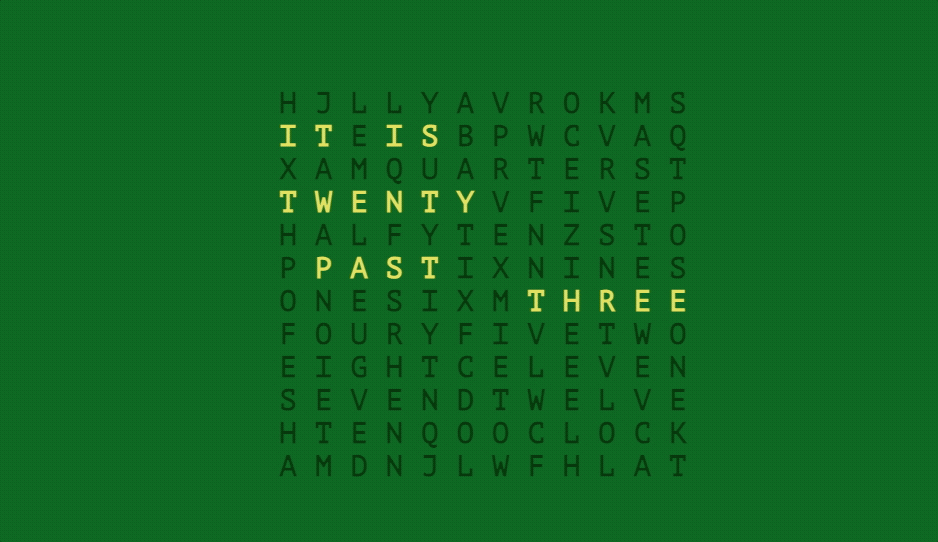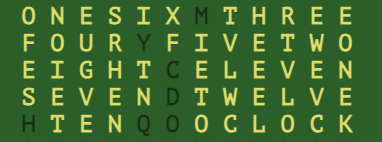# 纯 CSS 实现炫酷文本时钟

• 2023-11-21
广东
• 本文字数：2030 字

阅读完需：约 7 分钟• 整体文本布局呈正方形，包含需要高量的英文单词，但也包含一些不需要高亮的字母，仅是为了组合整个正方形布局

• 时间的跳动不是以一分钟级别的进行，而是以英文 5 分钟级别跳动

• 当前时间所需单词需要高亮

• 不需要使用 JS 定时器，仅做初始化时间获取

## 实现过程

### 界面布局<span class="hour h4">F O U R</span> Y <span class="hour h5">F I V E</span> <span class="hour h2">T W O</span><br/><span class="hour h8">E I G H T</span> C <span class="hour h11">E L E V E N</span><br/><span class="hour h7">S E V E N</span> D <span class="hour h0">T W E L V E</span><br/>

### CSS 样式

<span class="min m15">Q U A R T E R</span><span class="min m30">H A L F</span> <span class="before">T O</span><span class="after">P A S T</span>

### 动画实现

$current: #dddd55;$disable: rgba(0,0,0,0.5);$time: 3600s; 复制代码 然后通过@keyframes定义动画，这些动画控制文本颜色的变化，从而突出显示当前时间。例如： @keyframes m0m30 {}@keyframes m5m25m35m55 {}@keyframes m10m50 {}@keyframes m20m25m35m40 {}@keyframes m15m45 {}.m0, .m30 { animation: infinite m0m30 step-end$time;}.m5 {  animation: infinite m5m25m35m55 step-end $time;}.m15, .m45 { animation: infinite m15m45 step-end$time;}

$k: 0;@while$k < 60 {  .minute#{$k} {$l: 0;    @while $l < 60 { .m#{$l} {        animation-delay: - $time *$k / 60;      }      $l:$l + 5;    }        .before,    .after { animation-delay: -$time *$k / 60; }    .m30 { animation-delay: $time/2 -$time * $k / 60; }  @for$i from 0 through 11 {      &.hour#{$i} { @for$j from 0 through 11 {          .h#{$j} { animation-delay: (-$time*5/12 + $time*($j - $i)) - ($time * $k / 60); } } } } }$k: $k + 5;} 复制代码 在这个内部循环中，定义了一个类名为.m#{$l}的选择器，其中#{$l}会被替换为当前的$l值。然后，设置了animation-delay属性，其值为-$time *$k / 60。这样可以根据时间的变化来控制动画的延迟。小时方面生成了一个.h#{$j}的类名选择器，同样设置了animation-delay属性，其值为(-$time*5/12 + $time*($j - $i)) - ($time * \$k / 60)。这样可以根据小时和分钟的变化来控制时钟的动画延迟。

## 参考## 评论